CAT  >  Test: Time, Distance & Work

# Test: Time, Distance & Work

Test Description

## 30 Questions MCQ Test Topic-wise Past Year Questions for CAT | Test: Time, Distance & Work

Test: Time, Distance & Work for CAT 2023 is part of Topic-wise Past Year Questions for CAT preparation. The Test: Time, Distance & Work questions and answers have been prepared according to the CAT exam syllabus.The Test: Time, Distance & Work MCQs are made for CAT 2023 Exam. Find important definitions, questions, notes, meanings, examples, exercises, MCQs and online tests for Test: Time, Distance & Work below.
Solutions of Test: Time, Distance & Work questions in English are available as part of our Topic-wise Past Year Questions for CAT for CAT & Test: Time, Distance & Work solutions in Hindi for Topic-wise Past Year Questions for CAT course. Download more important topics, notes, lectures and mock test series for CAT Exam by signing up for free. Attempt Test: Time, Distance & Work | 30 questions in 50 minutes | Mock test for CAT preparation | Free important questions MCQ to study Topic-wise Past Year Questions for CAT for CAT Exam | Download free PDF with solutions
 1 Crore+ students have signed up on EduRev. Have you?
Test: Time, Distance & Work - Question 1

### One can use three different transports which move at 10, 20, and 30 kmph, respectively. To reach from A to B, Amal took each mode of transport 1/3 of his total journey time, while Bimal took each mode of transport 1/3 of the total distance. The percentage by which Bimal’s travel time exceeds Amal’s travel time is nearest to (2019)

Detailed Solution for Test: Time, Distance & Work - Question 1

Let the total journey time taken by Amal be 3t hours.
Hence, total distance = 10t + 20t + 30t = 60t kms Bimal took each mode of transport 1/3 of the total distance.
Therefore, total time taken by him =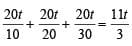hours
The percentage by which Bimal’s travel time exceeds Amal’s travel time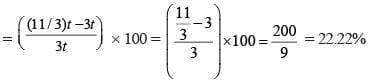Test: Time, Distance & Work - Question 2

### Two cars travel the same distance starting at 10:00 am and 11:00 am, respectively, on the same day. They reach their common destination at the same point of time. If the first car travelled for at least 6 hours, then the highest possible value of the percentage by which the speed of the second car could exceed that of the first car is (2019)

Detailed Solution for Test: Time, Distance & Work - Question 2

Let the distance of the common destination = d
and time taken by first car to reach the destination = t
then time taken by second car to reach the destination = t – 1.
∴ Speed of the first  car, S1 = d / t
and speed of the second car, S2 =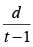Percentage increase in the speed of the second car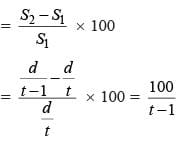It is given that t ≥ 6 ⇒ t – 1 ≥ 5
Now percentage increase in the speed of second car will be maximum when (t – 1) will be minimum i.e. 5.
∴ maximum percentage increase in the speed of the second car.
= 100 / 5 = 20

Test: Time, Distance & Work - Question 3

### In a race of three horses, the first beat the second by 11 metres and the third by 90 metres. If the second beat the third by 80 metres, what was the length, in metres, of the racecourse? (2019)

Detailed Solution for Test: Time, Distance & Work - Question 3

Let the first, second and third horses be A, B and C respectively. Also, let the length of the race course be x.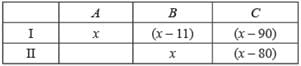Distance = Speed × Time
Now, DB = SB × t1
From first given condition, x – 11 = SB × t1 ...(i) and from second given condition, x = SB × t2 ...(ii)
Similarly, x – 90 = SC × t1 ...(iii)
and x –  80 = SC × t2 ...(iv)
Now dividing equation (i) by (iii), we get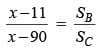...(v)
And dividing equation (ii) by (iv), we get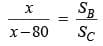From equation (v) and (vi), we get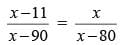⇒ x2 – 91x + 880 = x2 – 90x ⇒ x = 880

Test: Time, Distance & Work - Question 4

The wheels of bicycles A and B have radii 30 cm and 40 cm, respectively. While traveling a certain distance, each wheel of A required 5000 more revolutions than each wheel of B. If bicycle B traveled this distance in 45 minutes, then its speed, in km per hour, was

(2019)

Detailed Solution for Test: Time, Distance & Work - Question 4

Distance travelled by bicycle A in one revolution = 2πra = 2π × 30 = 60π cm
Distance travelled by bicycle B in one revolution = 2πrb = 2π × 40 = 80π cm
Let B makes ‘n’ revolutions to cover the distance. Then, A would make (n + 5000) revolution to cover the same distance.
∴ n × 80π = (n + 5000) × 60π ⇒ n = 15000
Distance travelled by B = n × 80π cm =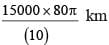= 12π km
Time taken by B = 45 min =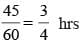Hence the speed of B =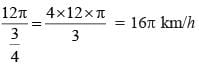Test: Time, Distance & Work - Question 5

At their usual efficiency levels, A and B together finish a task in 12 days. If A had worked half as efficiently as she usually does, and B had worked thrice as efficiently as he usually does, the task would have been completed in 9 days. How many days would A take to finish the task if she works alone at her usual efficiency?

(2019)

Detailed Solution for Test: Time, Distance & Work - Question 5

LCM of 9 and 12 = 36
Let the amount of work = 36 units
Let the amount of work done in one day by A and B with their normal efficiencies be x and y units respectively.
∴ (x + y) × 12 = 36 ⇒ x + y = 3 ⇒ y = 3 – x ...(i)
and (x/2 + 3y) × 9 = 36 ⇒ x/2 + 3y = 4 ⇒ x + 6y = 8 ...(ii)
Putting the value of y from eq. (i) into eq. (ii), we get
x + 6(3 – x) = 8 ⇒ –5x = 8 – 18 ⇒ x = 2
Therefore, A alone would take 36/x = 36/2 = 18 days to complete the work with her normal efficiency.

Test: Time, Distance & Work - Question 6

Three men and eight machines can finish a job in half the time taken by three machines and eight men to finish the same job. If two machines can finish the job in 13 days, then how many men can finish the job in 13 days?

(2019)

Detailed Solution for Test: Time, Distance & Work - Question 6

Let one machine completes 1 unit of work per day.
Since, two machines can finish the job in 13 days
∴ Amount of work = 2 × 1 × 13 = 26 units.
Also, let a man completes M units of work per day.
From the given conditions 3M + 8 × 1 = 2(8M + 3 × 1) ⇒ M = 2 / 13 units
Let it require ‘x’ number of men to complete the work in 13 days.
xM × 13 = 26 units ⇒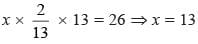hence, required number of men = 13.

Test: Time, Distance & Work - Question 7

John jogs on track A at 6 kmph and Mary jogs on track B at 7.5 kmph. The total length of tracks A and B is 325 metres. While John makes 9 rounds of track A, Mary makes 5 rounds of track B. In how many seconds will Mary make one round of track A?

(2019)

Detailed Solution for Test: Time, Distance & Work - Question 7

Speed of John = 6 kmph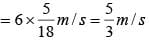Speed of Mary = 7.5 kmph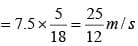Let the track length of A and B be x and y respectively
Given, x + y = 325  ...(i)
Time taken by John to cover one round of track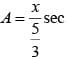Therefore, time taken to cover 9 rounds of track A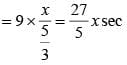Time taken by Mary to cover one round of track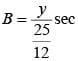Therefore, time taken to cover 5 rounds of track B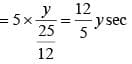According to the question,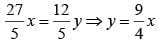..(ii)
Put the value of y in (i)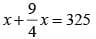⇒ 13x = 1300 ⇒ x = 100
∴ Time taken by Mary to cover one round of track A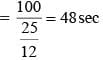Test: Time, Distance & Work - Question 8

Anil alone can do a job in 20 days while Sunil alone can do it in 40 days. Anil starts the job, and after 3 days, Sunil joins him. Again, after a few more days, Bimal joins them and they together finish the job. If Bimal has done 10% of the job, then in how many days was the job done?

(2019)

Detailed Solution for Test: Time, Distance & Work - Question 8

LCM of 20 and 40 = 40
Let the work be of 40 units
Amount of work done by Anil in one day = 40/20 = 2 units
Amount of work done by Sunil in one day = 4
40 / 40 = 1 units
Bimal does 10% work i.e. 4 units work
Rest 40 – 4 = 36 units is done by Anil and Sunil.
Let Anil took x days. Therefore, Sunil took (x – 3) days.
Therefore 2 × x + 1 × (x – 3) = 36 ⇒ x = 13 days.
Hence, the job was done in 13 days.

Test: Time, Distance & Work - Question 9

A cyclist leaves A at 10 am and reaches B at 11 am. Starting from 10:01 am, every minute a motor cycle leaves A and moves towards B. Forty-five such motor cycles reach B by 11 am. All motor cycles have the same speed. If the cyclist had doubled his speed, how many motor cycles would have reached B by the time the cyclist reached B?

(2019)

Detailed Solution for Test: Time, Distance & Work - Question 9

Time taken by cyclist to cover the distance AB = 60 min Given, starting from 10:01 am, every minute a motor cycle leaves A and moves towards B.
Forty-five such motor cycles reach B by 11 am.
Also, the speed of all the motor cycles is same This means that the 45th motor cycle, which started at 10:45 am, reached B exactly at 11 am, Rest all reached B some time before B.
Therefore, each motor cycle takes 15 min to cover the distance AB.
Now, if the cyclist doubles his speed, then he will reach B in 30 min i.e. at 10:30 am.
So, the 15th motor cycle (started at 10:15 am from A) would be the last motor cycle that reach to point B at 10:30 am.
Hence, when the cyclist had doubled his speed, then there will be 15 motor cycles would have reached B by the time the cyclist reached B.

Test: Time, Distance & Work - Question 10

Two ants A and B start from a point P on a circle at the same time, with A moving clock-wise and B moving anticlockwise. They meet for the first time at 10:00 am when A has covered 60% of the track. If A returns to P at 10:12 am, then B returns to P at

(2019)

Detailed Solution for Test: Time, Distance & Work - Question 10

Let the track length be 10x
When they meet at 10 am, ant A travelled 6x of the distance and ant B travelled 4x of the distance.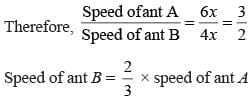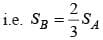The distance covered by ant A from meeting point to point P was 4x. Similarly, the distance covered by ant B from meeting point to point P was 6x.
Given, ant A took 12 min to reach P.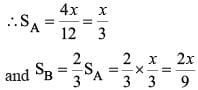Time taken by ant B to travelled the 6x distance to reach at P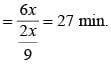Therefore ant B reaches P at 10:27 am.

Test: Time, Distance & Work - Question 11

John gets ₹ 57 per hour of regular work and ₹ 114 per hour of overtime work. He works altogether 172 hours and his income from overtime hours is 15% of his income from regular hours. Then, for how many hours did he work overtime?

(2019)

Detailed Solution for Test: Time, Distance & Work - Question 11

Let the number of hours for regular and overtime work be x and y respectively.
∴ x + y = 172 ...(i)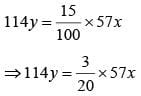⇒ 40y = 3x
⇒ 3x – 40y = 0 ...(ii)
On solving equations (i) and (ii), we get x = 160 and y = 12
∴ overtime work = 12 hours

Test: Time, Distance & Work - Question 12

Humans and robots can both perform a job but at different efficiencies. Fifteen humans and five robots working together take thirty days to finish the job, whereas five humans and fifteen robots working together take sixty days to finish it. How many days will fifteen humans working together (without any robot) take to finish it?

(2018)

Detailed Solution for Test: Time, Distance & Work - Question 12

Let the rates of work of each human and each robot be H  units per day and R units per day respectively.
∴ 15H + 5R = 1 / 30 ...(i)
and 5H + 15R = 1 / 60 ..(ii)
⇒ 3 (i) – (ii) ⇒ 40H = 1 / 12
H = 1 / 480
In a day, 15 humans can complete 15H i.e. 1/32th of the job.
∴ 15 humans can complete the job in 32 days.

Test: Time, Distance & Work - Question 13

A tank is fitted with pipes, some filling it and the rest draining it. All filling pipes fill at the same rate, and all draining pipes drain at the same rate. The empty tank gets completely filled in 6 hours when 6 filling and 5 draining pipes are on, but this time becomes 60 hours when 5 filling and 6 draining pipes are on. In how many hours will the empty tank get completely filled when one draining and two filling pipes are on?

(2018)

Detailed Solution for Test: Time, Distance & Work - Question 13

Let the rates at which each filling pipe and each draining pipe works be F units/hr and D units/hr.
∴ 6F – 5D = 1 / 6 ..(i)
and 5F – 6D = 1 / 60 ..(ii)
on solving (i) and (ii), we get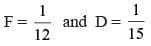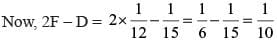Hence one draining and two filling pipes can fill the tank in 10 hours.

Test: Time, Distance & Work - Question 14

When they work alone, B needs 25% more time to finish a job than A does. They two finish the job in 13 days in the following manner: A works alone till half the job is done, then A and B work together for four days, and finally B works alone to complete the remaining 5% of the job. In how many days can B alone finish the entire job?

(2018)

Detailed Solution for Test: Time, Distance & Work - Question 14

Let the time taken by A to finish the job be “a” days.
Time taken by B to finish the job = 5a/4 days
Part of the job completed when A and B worked together for 4 days =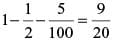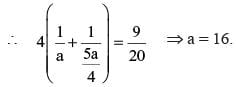Time taken by B alone to complete the entire job = 5a/4 = 20 days.

Test: Time, Distance & Work - Question 15

Point P lies between points A and B such that the length of BP is thrice that of AP. Car 1 starts from A and moves towards B. Simultaneously, car 2 starts from B and moves towards A. Car 2 reaches P one hour after car 1 reaches P. If the speed of car 2 is half that of car 1, then the time, in minutes, taken by car 1 in reaching P from A is

(2018)

Detailed Solution for Test: Time, Distance & Work - Question 15

Let the time taken for car 1 to reach P from A be x hours.
Speed of car 1=AP/x BP = 3AP.
Car 2 starts from B to A and reaches P one hour after car 1 reaches P.
Speed of car 2 =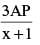Now speed of car 2 =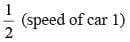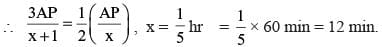∴  Time taken for car 1 to reach P from A is 12 min.

Test: Time, Distance & Work - Question 16

Train T leaves station X for station Y at 3 pm. Train S, traveling at three quarters of the speed of T, leaves Y for X at 4 pm. The two trains pass each other at a station Z, where the distance between X and Z is three-fifths of that between X and Y. How many hours does train T take for its journey from X to Y?

(2018)

Detailed Solution for Test: Time, Distance & Work - Question 16

Let the time taken by S to reach Z be t hours.
Let the speed of T be St. Distance between X and Z is 3/5 of the distance between X and Y.
XZ : ZY = 3 : 2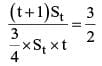t = 8
S takes 8 hours to cover YZ.
T would take 8 × (3/4) i.e. 6 hours to cover ZY.
T would take t + 1 i.e. 9 hours to cover XZ.
T would take 15 hours to reach Y.

Test: Time, Distance & Work - Question 17

The distance from A to B is 60 km. Partha and Narayan start from A at the same time and move towards B. Partha takes four hours more than Narayan to reach B. Moreover, Partha reaches the mid-point of A and B two hours before Narayan reaches B. The speed of Partha, in km per hour, is

(2018)

Detailed Solution for Test: Time, Distance & Work - Question 17

Let the speeds of Partha and Narayan be Sp and Sn respectively.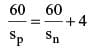...(i)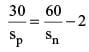...(ii)
Subtraction, equation (ii) from (i), we get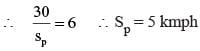Test: Time, Distance & Work - Question 18

A man travels by a motor boat down a river to his office and back. With the speed of the river unchanged, if he doubles the speed of his motor boat, then his total travel time gets reduced by 75%. The ratio of the original speed of the motor boat to the speed of the river is

(2017)

Detailed Solution for Test: Time, Distance & Work - Question 18

Let the speed of the boat in still water and the speed of the river be u and v respectively
u = x
v = y
According to question,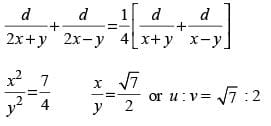Test: Time, Distance & Work - Question 19

There are three cities A, B and C, not on the same straight road. Two buses P and Q start simultaneously from A and B respectively towards C. By the time Q reaches C, P is exactly halfway to C. Immediately after Q reaches C, it starts travelling towards A and it crosses P at a point 165 km from A. The ratio of the speeds of P and Q is 3 : 5 Assume that the roads joining A to C, B to C and B to A are all straight roads. If B is twice as far from A as it is from C and P would take to cover the distance from A to B, how much time would Q take to cover the distance from C to A?

(2016)

Detailed Solution for Test: Time, Distance & Work - Question 19

Let BC = 5 k
Given, by the time Q reaches C, P was halfway to C, i.e., AC/2 = 3k and AC = 6k.
As Q met P, 165 km away from A, the distance to the meeting point from A is 3k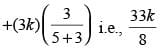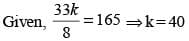∴ Distance between A and C is 240 km and that between B and C is 200 km.
From the data, as distance between A and B is twice that between B and C, it is 400 km.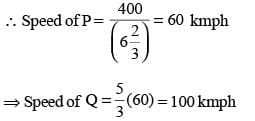∴ Time taken by Q to reach C from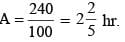Test: Time, Distance & Work - Question 20

There is a string of length 100 m running from east to west. 1000 ants are dropped onto the string. Assume that each ant lands on the string facing either the east or the west direction. As soon as they land, each ant starts moving in the direction which is being faced by it at 50 m/ min till it falls off the string. But if an ant collides with another ant coming from the opposite direction, both of them reverse their directions and proceed to move now in the opposite directions. Ants fall only at either of the ends of the string. What is the minimum time by which the string is definitely free of ants?

(2015)

Detailed Solution for Test: Time, Distance & Work - Question 20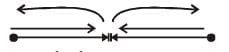Let's take there are two ants.
Considering worst possible case we can see easily the required time is same as the time taken by an ant to reach one extreme point to another extreme point.
Which will be same when there are 1000 ants.
i.e.maximum time =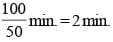Test: Time, Distance & Work - Question 21

P, Q and R start walking from the same point. P and Q start at 1 PM and R starts at 3 PM. R takes over P at 5 PM and then doubles his speed and takes over Q after another hour. What is the ratio of speed of A and speed of B?

(2014)

Detailed Solution for Test: Time, Distance & Work - Question 21

The distance covered by R in 2 hours (3 PM to 5 PM) is same as the distance covered by P in 4 hours (1 PM to 5 PM).
Ratio of speeds of P and R = 1 : 2.
Now, R takes over Q in another hour by doubling his speed, so R has covered a total distance in 3 hours (3 PM to 6 PM) which he would have covered in 4 hours without changing his speed.
To cover the same distance, a takes 5 hours (1 PM to 6 PM) Ratio of speed of Q and R = 4: 5
⇒ Ratio of speed of P and Q = 5: 8.

Test: Time, Distance & Work - Question 22

Once upon a time, the king of a jungle planned a 2-kilometre race between a rabbit and a tortoise. Soon after the start of the race, the rabbit took a huge lead. On realizing that even after taking a nap of ‘t’ min, he would beat the tortoise by 13 min, he stooped under a tree and went to sleep. Meanwhile the tortoise kept walking. When the rabbit woke up, he realized that he had slept for (14 + t) min, and immediately started running towards the target at a speed 3/2 times his original speed. The race eventually ended in a dead heat. If the ratio of the original speed of the rabbit to that of the tortoise was 6 : 1 and the rabbit overstretched his nap by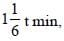then how long did the tortoise take to complete the race?

(2013)

Detailed Solution for Test: Time, Distance & Work - Question 22

The rabbit overstretched his sleeping time by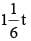min.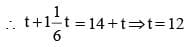Let the speed (in km/min) of the rabbit and tortoise be 6x and x respectively.
Had the rabbit not overstretched his hap, he would have beaten the tortoise by 13 min.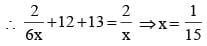Hence the time taken by the tortoise to complete the race =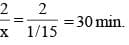Test: Time, Distance & Work - Question 23

Anushka and Anirudh working together can complete a piece of work in 20 days. They started the work together, but Anushka left after x days and Anirudh finished the remaining work in the next x/2 days. Had Anushka left after 3x/4 days, Anirudh would have taken x days to finish the remaining work. Find the ratio of the efficiency of Anushka to that of Anirudh.

(2013)

Detailed Solution for Test: Time, Distance & Work - Question 23

Let the efficiencies of Anirudh and Anushka be 'a' and 'b' units/day respectively.
according to question,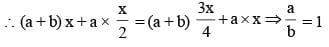So, the required ratio is 1 : 1.

Test: Time, Distance & Work - Question 24

During his trip to England, Mr. Clockilal, a horologist, decided to visit ‘The Cuckooland Museum’ dedicated to the exhibition of cuckoo clocks. He entered the museum between 12 noon and 1 p.m. The moment he entered the museum, he observed that the angle between the minute hand and the hour hand of one of the clocks was 20°. While leaving the museum, he observed that the angle between the minute hand and the hour hand of the same clock was 220°. If he spent more than 3 hours and less than 4 hours in the museum, then how much time did he exactly spend in the museum?

(2013)

Detailed Solution for Test: Time, Distance & Work - Question 24

Let the time at which Mr. Clockilal entered the museum be 'm' minutes past 12 noon.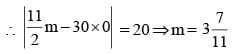As he spent more than 3 hours and less than 4 hours in the museum, and the angle between the minute and hour hands at the time of leaving was 220°, he must have left the museum between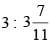p.m. and 4 p.m.
Let the time at which he left the museum be 'n' minutes past 3 pm.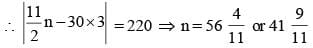Hence, the time spent by Mr. Clcckilal in the museum was either 3 hours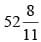minutes or 3 hours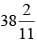minutes.

Test: Time, Distance & Work - Question 25

The radius of the cross-sections of pipes P1 and P2 are 7 m and 14 m respectively. Water flows through P1 at a constant rate of 10 m/s and it can alone fill a tank in 2 hours. If P1 is used as the inlet pipe and P2 as the outlet pipe then together they fill the tank in 4 hours. What is the rate of water flow (in m/s) through P2?

(2012)

Detailed Solution for Test: Time, Distance & Work - Question 25

Area of cross section for P1 pipe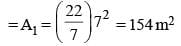Volume of water flowing through P1 in one second = 154 × 10 = 1540 m3
Volume of tank = V = 1540 × 2 × 3600 = 11088 × 103 m3
Area of cross section for P2 pipe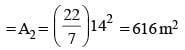Let the rate of water flowing through P2 = s m/s
Volume of water flowing through P2 in one second = 616s m3
Volume of water flow in the tank when P1 is used as inlet and P2 is used as outlet pipe: (1540 – 616s)
Time taken to fill = 4 hours = 4 × 3600 seconds
So, (1540 – 616s) × 4 × 3600 = V
⇒ (1540 – 616s) × 4 × 3600 = 11088 × 103
or 1540 – 616s = 770
or s = 1.25 m/s
∴  Rate of water flow through P2 = 1.25 m/s

Test: Time, Distance & Work - Question 26

A train crosses a platform 100 metres long in 60 seconds at a speed of 45 km per hour. The time taken by the train to cross an electric pole, is

(2011)

Detailed Solution for Test: Time, Distance & Work - Question 26

Let the length of train = x metres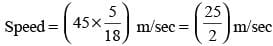Distance covered in crossing the platform = (x + 100) m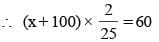or   2x + 200 = 1500 or x = 650
Now, time taken to cross the pole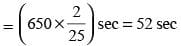Test: Time, Distance & Work - Question 27

In a 400 meter race around a circular stadium having a circumference of 1000 meters, the fastest runner and the slowest runner reach the same point at the end of the 5th minute, for the first time after the start of the race. All the runners have the same starting point and each runner maintains a uniform speed throughout the race. If the fastest runner runs at twice the speed of the slowest runner, what is the time taken by the fastest runner to finish the race?

(2011)

Detailed Solution for Test: Time, Distance & Work - Question 27

Let the slowest runner covers a distance of x ms. from the starting point.
Then the fastest runner will cover a distance of 1000 + x from the starting point.
Let the speed of the slowest runner be y.
Then, speed of the fastest runner will be 2y.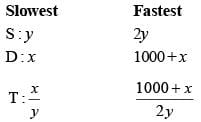But time taken for both is same .
Hence,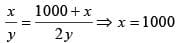∴ Fastest runner completes a distance of 2000m in 5 mins.
∴ Fastest runner can complete a distance of 4000m in 10 min.

Test: Time, Distance & Work - Question 28

A ship 55 kms. from the shore springs a leak which admits 2 tones of water in 6 min ; 80 tones would suffer to sink her, but the pumps can throw out 12 tones an hour. Find the average rate of sailing that she may just reach the shore as she begins to sink.

(2011)

Detailed Solution for Test: Time, Distance & Work - Question 28

= 2/6 tonnes / min. = 1/3 tonnes/ min
Rate of pumping out of water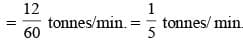Rate of accumulation =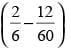tonnes / min.
Time to accumulate 80 tonnes of water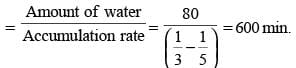= 10 hours
∴ Average sailing rate so as avoid sinking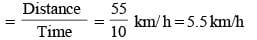Test: Time, Distance & Work - Question 29

It takes 6 technicians a total of 10 hours to build a new server from direct computer, with each working at the same rate. If six technicians start to build the server at 11 : 00 am, and one technician per hour is added beginning at 5 :00 pm, at what time will the server be completed?

(2010)

Detailed Solution for Test: Time, Distance & Work - Question 29

Total time taken to build the server = 60 man hours.
6 of them starts at 11 : 00 am and works till 5 pm They will complete 6 × 6 = 36 man hours of work.
At 5 pm they will have 24 more man hours of work to complete.
Between 5 pm and 6 pm they will complete 7 man hours.
Between 6 pm and 7 pm they will complete 8 man hours.
Between 7 pm and 8 pm they will complete 9 man hours.
So, totally they will complete 36 + 7 + 8 + 9 = 60 man hours by 8 pm.

Test: Time, Distance & Work - Question 30

A watch, which gains time uniformly, was 5 minutes behind the correct time when it showed 11:55 AM on Monday. It was 10 minutes ahead of the correct time when it showed 06:10 PM on the next day. When did the watch show the correct time?

(2010)

Detailed Solution for Test: Time, Distance & Work - Question 30

The watch gains (5 + 10) = 15 min in 30 hours (12 Noon to 6 PM next day).
This means that it will show the correct time when it gains 5 min in 10 hours or at 10 PM on Monday.

## Topic-wise Past Year Questions for CAT

43 docs|31 tests
 Use Code STAYHOME200 and get INR 200 additional OFF Use Coupon Code
Information about Test: Time, Distance & Work Page
In this test you can find the Exam questions for Test: Time, Distance & Work solved & explained in the simplest way possible. Besides giving Questions and answers for Test: Time, Distance & Work, EduRev gives you an ample number of Online tests for practice

## Topic-wise Past Year Questions for CAT

43 docs|31 tests

### How to Prepare for CAT

Read our guide to prepare for CAT which is created by Toppers & the best Teachers